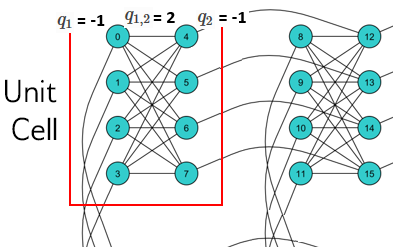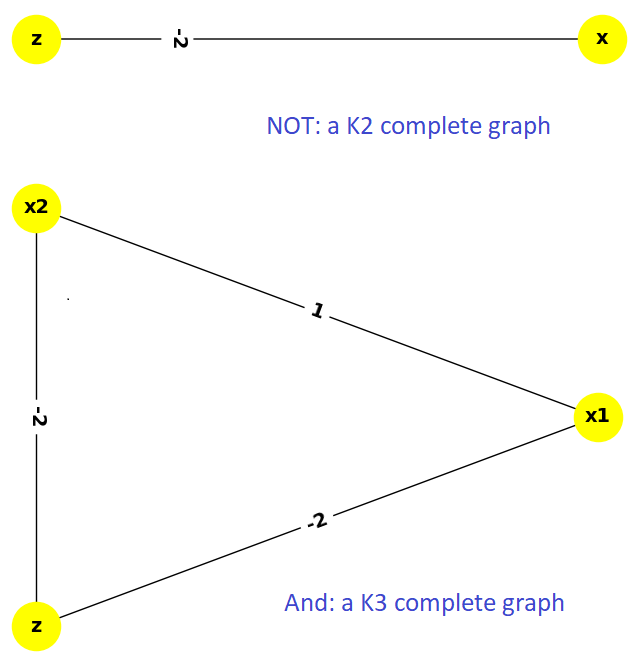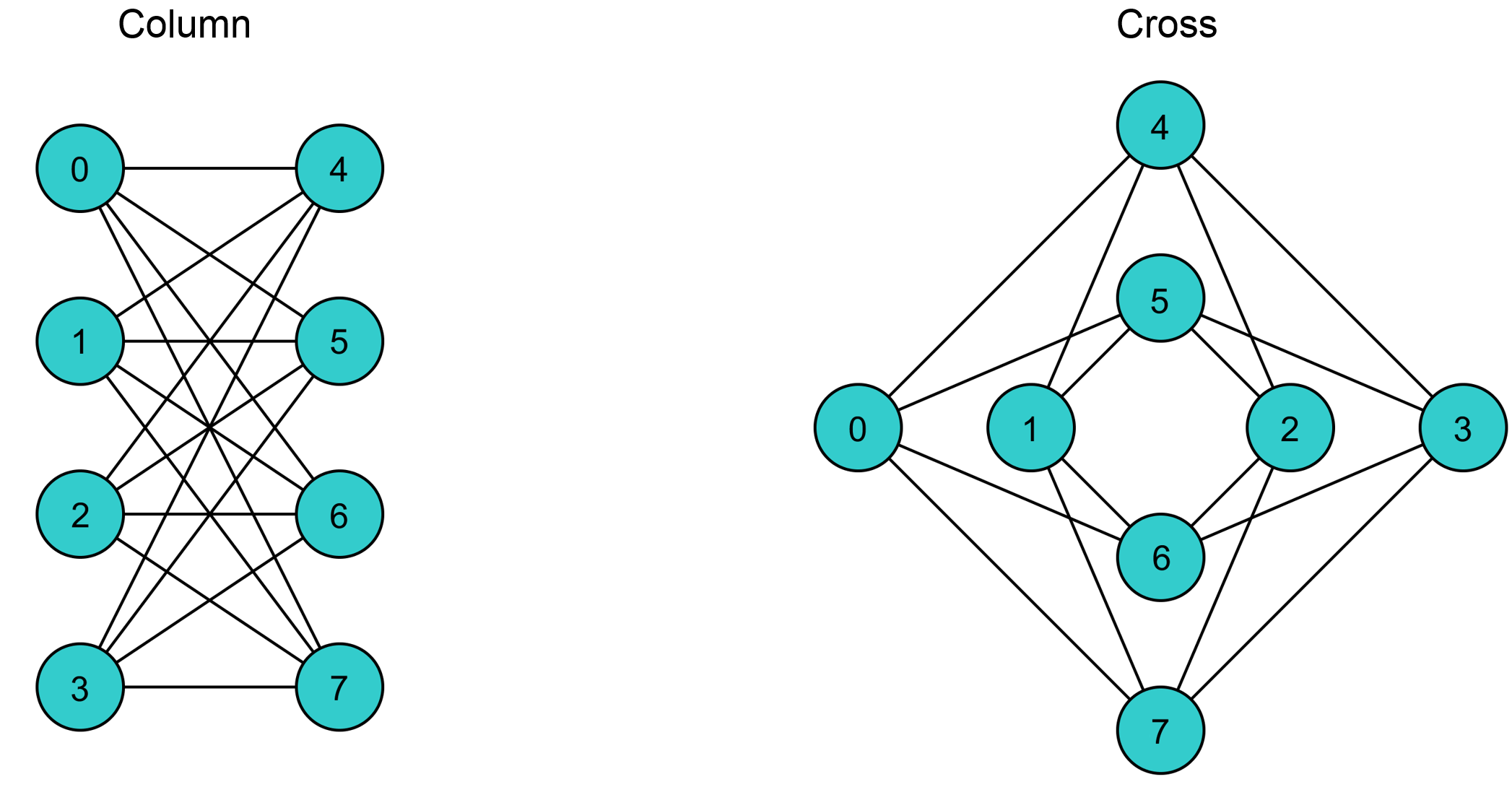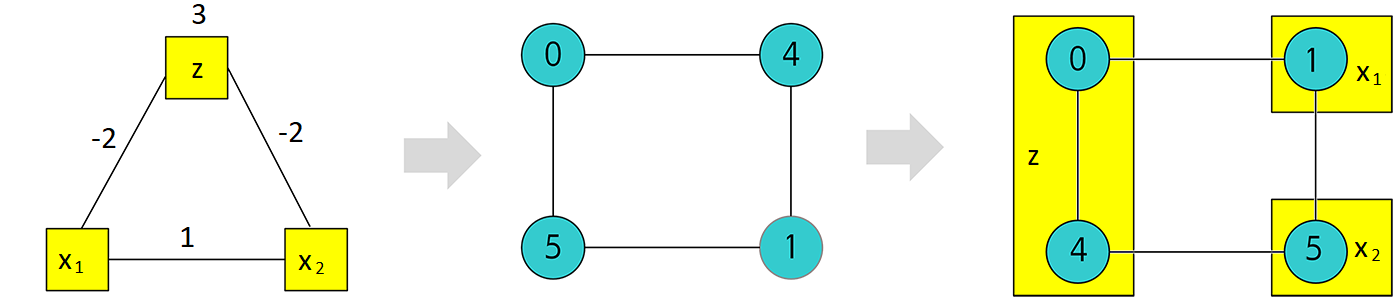# Boolean AND Gate¶

This example solves a simple problem of a Boolean AND gate on a D-Wave system to demonstrate programming the underlying hardware more directly; in particular, minor-embedding a chain.

Other examples demonstrate more advanced steps that are typically needed for solving actual problems.

## Example Requirements¶

To run the code in this example, the following is required.

If you installed dwave-ocean-sdk and ran dwave config create, your installation should meet these requirements.

## Solution Steps¶

Section Solving Problems on a D-Wave System describes the process of solving problems on the quantum computer in two steps: (1) Formulate the problem as a binary quadratic model (BQM) and (2) Solve the BQM with a D-wave system or classical sampler. In this example, we mathematically formulate the BQM and use Ocean tools to solve it on a D-Wave system.

## Formulate the AND Gate as a BQM¶

Ocean tools can automate the representation of logic gates as a BQM, as demonstrated in the Multiple-Gate Circuit example. The Boolean NOT Gate example presents a mathematical formulation of a BQM for a Boolean gate in detail. Here we briefly repeat the steps of mathematically formulating a BQM while adding details on the underlying physical processes.

A D-Wave quantum processing unit (QPU) is a chip with interconnected qubits; for example, a D-Wave 2000Q has up to 2048 qubits connected in a Chimera topology. Programming it consists mostly of setting two inputs:

• Qubit bias weights: control the degree to which a qubit tends to a particular state.
• Qubit coupling strengths: control the degree to which two qubits tend to the same state.

The biases and couplings define an energy landscape, and the D-Wave quantum computer seeks the minimum energy of that landscape. Once you express your problem in a formulation such that desired outcomes have low energy values and undesired outcomes high energy values, the D-Wave system solves your problem by finding the low-energy states.

  This formulation, called an objective function, corresponds to the Ising model traditionally used in statistical mechanics: given $$N$$ variables $$s_1,...,s_N$$, corresponding to physical Ising spins, where each variable $$s_i$$ can have values $$-1$$ or $$+1$$, the system energy for an assignment of values is, $E(\pmb{s}|\pmb{h},\pmb{J}) = \left\{ \sum_{i=1}^N h_i s_i + \sum_{i Here we use another binary quadratic model (BQM), the computer-science equivalent of the Ising model, the QUBO: given $$M$$ variables $$x_1,...,x_N$$, where each variable $$x_i$$ can have binary values $$0$$ or $$1$$, the system tries to find assignments of values that minimize \[E(q_i, q_{i,j}; x_i) = \sum_i^N q_ix_i + \sum_{i<j}^N q_{i,j}x_i x_j,$

where $$q_i$$ and $$q_{i,j}$$ are configurable (linear and quadratic) coefficients. To formulate a problem for the D-Wave system is to program $$q_i$$ and $$q_{i,j}$$ so that assignments of $$x_1,...,x_N$$ also represent solutions to the problem.

### AND as a Penalty Function¶

This example represents the AND operation, $$z \Leftrightarrow x_1 \wedge x_2$$, where $$x_1, x_2$$ are the gate’s inputs and $$z$$ its output, using a penalty function:

$x_1 x_2 - 2(x_1+x_2)z +3z.$

This penalty function represents the AND gate in that for assignments of variables that match valid states of the gate, the function evaluates at a lower value than assignments that would be invalid for the gate. Therefore, when the D-Wave system minimizes a BQM based on this penalty function, it finds those assignments of variables that match valid gate states.

You can verify that this penalty function represents the AND gate in the same way as was done in the Boolean NOT Gate example. See the D-Wave Problem-Solving Handbook for more information about penalty functions in general, and penalty functions for representing Boolean operations in particular.

### Formulating the Problem as a QUBO¶

For this example, the penalty function is quadratic, and easily reordered in the familiar QUBO formulation:

$E(q_i, q_{i,j}; x_i) = 3x_3 + x_1x_2 - 2x_1x_3 - 2x_2x_3$

where $$z=x_3$$ is the AND gate’s output, $$x_1, x_2$$ the inputs, linear coefficients are $$q_1=3$$, and quadratic coefficients are $$q_{1,2}=1, q_{1,3}=-2, q_{2,3}=-2$$. The coefficients matrix is,

$\begin{split}Q = \begin{bmatrix} 0 & 1 & -2\\ & 0 & -2\\ & & 3 \end{bmatrix}\end{split}$

See the Getting Started with the D-Wave System and D-Wave Problem-Solving Handbook books for more information about formulating problems as QUBOs.

The line of code below sets the QUBO coefficients for this AND gate.

>>> Q = {('x1', 'x2'): 1, ('x1', 'z'): -2, ('x2', 'z'): -2, ('z', 'z'): 3}


## Solve the Problem by Sampling: Automated Minor-Embedding¶

For reference, we first solve with the same steps used in the Boolean NOT Gate example before solving again while manually controlling additional parameters.

Again we use sampler DWaveSampler() from Ocean software’s dwave-system and its EmbeddingComposite() composite to minor-embed our unstructured problem (variables x1, x2, and z) on the sampler’s graph structure (the QPU’s numerically indexed qubits).

The next code sets up a D-Wave system as the sampler.

Note

In the code below, replace sampler parameters in the third line. If you configured a default solver, as described in Using a D-Wave System, you should be able to set the sampler without parameters as sampler = DWaveSampler(). You can see this information by running dwave config inspect in your terminal.

>>> from dwave.system.samplers import DWaveSampler
>>> from dwave.system.composites import EmbeddingComposite
>>> sampler = DWaveSampler(endpoint='https://URL_to_my_D-Wave_system/', token='ABC-123456789012345678901234567890', solver='My_D-Wave_Solver')
>>> sampler_embedded = EmbeddingComposite(sampler)


As before, we ask for 5000 samples.

>>> response = sampler_embedded.sample_qubo(Q, num_reads=5000)
>>> for datum in response.data(['sample', 'energy', 'num_occurrences']):
...    print(datum.sample, "Energy: ", datum.energy, "Occurrences: ", datum.num_occurrences)
...
{'x1': 1, 'x2': 0, 'z': 0} Energy:  0.0 Occurrences:  1009
{'x1': 1, 'x2': 1, 'z': 1} Energy:  0.0 Occurrences:  1452
{'x1': 0, 'x2': 0, 'z': 0} Energy:  0.0 Occurrences:  1292
{'x1': 0, 'x2': 1, 'z': 0} Energy:  0.0 Occurrences:  1246
{'x1': 0, 'x2': 1, 'z': 0} Energy:  0.0 Occurrences:  1


All the returned samples from this execution represent valid value assignments for an AND gate, and minimize (are low-energy states of) the BQM.

Note that the last line of output from this execution shows a single sample that seems identical to the line above it. The next section addresses that.

## Solve the Problem by Sampling: Non-automated Minor-Embedding¶

This section looks more closely into minor-embedding. Above and in the Boolean NOT Gate example, dwave-system EmbeddingComposite() composite abstracted the minor-embedding.

### Minor-Embedding a NOT Gate¶

For simplicity, we first return to the NOT gate. The Boolean NOT Gate example found that a NOT gate can be represented by a BQM in QUBO form with the following coefficients:

>>> Q_not = {('x', 'x'): -1, ('x', 'z'): 2, ('z', 'x'): 0, ('z', 'z'): -1}


Minor embedding maps the two problem variables x and z to the indexed qubits of the D-Wave QPU. Here we do this mapping ourselves.

The next line of code looks at properties of the sampler. We select the first node, which on a QPU is a qubit, and print its adjacent nodes, i.e., coupled qubits.

>>> print(sampler.adjacency[sampler.nodelist])
{128, 4, 5, 6, 7}


For the D-Wave system the above code ran on, we see that the first available qubit is adjacent to qubit 4 and four others.

We can map the NOT problem’s two linear coefficients and single quadratic coefficient, $$q_1=q_2=-1$$ and $$q_{1,2}=2$$, to biases on qubits 0 and 4 and coupling (0, 4). The figure below shows a minor embedding of the NOT gate into a D-Wave 2000Q QPU unit cell (four horizontal qubits connected to four vertical qubits via couplers).A NOT gate minor embedded into the topmost left unit cell of a D-Wave 2000Q QPU. Variables $$x_1,x_2$$ are minor embedded as qubits 0 and 4 (blue circles). Biases $$q_1,q_2=-1,-1$$ and coupling strength $$q_{1,2}=2$$ are also shown.

The following code uses the FixedEmbeddingComposite composite to manually minor-embed the problem. Its last line prints a confirmation that indeed the two selected qubits are adjacent (coupled).

>>> from dwave.system.composites import FixedEmbeddingComposite
>>> sampler_embedded = FixedEmbeddingComposite(sampler, {'x': , 'z': })
{'x': {'z'}, 'z': {'x'}}


As before, we ask for 5000 samples.

>>> response = sampler_embedded.sample_qubo(Q_not, num_reads=5000)
>>> for datum in response.data(['sample', 'energy', 'num_occurrences']):
...    print(datum.sample, "Energy: ", datum.energy, "Occurrences: ", datum.num_occurrences)
...
{'x': 0, 'z': 1} Energy:  -1.0 Occurrences:  2520
{'x': 1, 'z': 0} Energy:  -1.0 Occurrences:  2474
{'x': 0, 'z': 0} Energy:  0.0 Occurrences:  4
{'x': 1, 'z': 1} Energy:  0.0 Occurrences:  2


### From NOT to AND: an Important Difference¶

• The BQM for a NOT gate, $$-x -z + 2xz$$, can be represented by a fully connected $$K_2$$ graph: its linear coefficients are weights of the two connected nodes with the single quadratic coefficient the weight of its connecting edge.
• The BQM for an AND gate, $$3z + x_1x_2 - 2x_1z - 2x_2z$$, needs a $$K_3$$ graph.NOT gate $$K_2$$ complete graph (top) versus AND gate $$K_3$$ complete graph (bottom.)

We saw above how to minor-embed a $$K_2$$ graph on a D-Wave system. To minor-embed a fully connected $$K_3$$ graph requires chaining qubits.

### Minor-Embedding an AND Gate¶

To understand how a $$K_3$$ graph fits on the Chimera topology of the QPU, look at the Chimera unit cell structure shown below. You cannot connect 3 qubits in a closed loop. However, you can make a closed loop of 4 qubits using, say, qubits 0, 1, 4, and 5.Chimera unit cell illustrated in two layouts.

To fit the 3-qubit loop into a 4-sided structure, create a chain of 2 qubits to represent a single variable. For example, chain qubit 0 and qubit 4 to represent variable $$z$$.Embedding a $$K_3$$ graph into Chimera by using a chain.

The strength of the coupler between qubits 0 and 4, which represents variable $$z$$, must be set to correlate the qubits strongly, so that in most solutions they have a single value for $$z$$. (Remember the output in the Solve the Problem by Sampling: Automated Minor-Embedding section with its identical two last lines? This was likely due to the qubits in a chain taking different values.)

The code below uses Ocean’s dwave-system FixedEmbeddingComposite() composite for manual minor-embedding. Its last line prints a confirmation that indeed all three variables are connected. (coupled).

>>> from dwave.system.composites import FixedEmbeddingComposite
>>> embedding = {'x1': {1}, 'x2': {5}, 'z': {0, 4}}
>>> sampler_embedded = FixedEmbeddingComposite(sampler, embedding)
{'x1': {'x2', 'z'}, 'x2': {'x1', 'z'}, 'z': {'x1', 'x2'}}


>>> Q = {('x1', 'x2'): 1, ('x1', 'z'): -2, ('x2', 'z'): -2, ('z', 'z'): 3}
>>> for datum in response.data(['sample', 'energy', 'num_occurrences']):
...    print(datum.sample, "Energy: ", datum.energy, "Occurrences: ", datum.num_occurrences)
...
{'z': 0, 'x1': 1, 'x2': 0} Energy:  0.0 Occurrences:  1088
{'z': 0, 'x1': 0, 'x2': 1} Energy:  0.0 Occurrences:  1806
{'z': 1, 'x1': 1, 'x2': 1} Energy:  0.0 Occurrences:  1126
{'z': 0, 'x1': 0, 'x2': 0} Energy:  0.0 Occurrences:  977
{'z': 1, 'x1': 0, 'x2': 1} Energy:  1.0 Occurrences:  2
{'z': 1, 'x1': 0, 'x2': 1} Energy:  1.0 Occurrences:  1


For comparison, the following code purposely weakens the chain strength (strength of the coupler between qubits 0 and 4, which represents variable $$z$$). The first line prints the range of values available for the D-Wave system this code is executed on. By default, FixedEmbeddingComposite() used the maximum chain strength, which is 2. By setting it to a low value of 0.25, the two qubits are not strongly correlated and the result is that many returned samples represent invalid states for an AND gate.

>>> print(sampler.properties['extended_j_range'])
[-2.0, 1.0]
>>> sampler_embedded = FixedEmbeddingComposite(sampler, embedding)
>>> response = sampler_embedded.sample_qubo(Q, num_reads=5000, chain_strength=0.25)
>>> for datum in response.data(['sample', 'energy', 'num_occurrences']):
...    print(datum.sample, "Energy: ", datum.energy, "Occurrences: ", datum.num_occurrences)
...
{'z': 0, 'x1': 1, 'x2': 0} Energy:  0.0 Occurrences:  690
{'z': 0, 'x1': 0, 'x2': 1} Energy:  0.0 Occurrences:  936
{'z': 1, 'x1': 1, 'x2': 1} Energy:  0.0 Occurrences:  573
{'z': 0, 'x1': 0, 'x2': 0} Energy:  0.0 Occurrences:  984
{'z': 1, 'x1': 1, 'x2': 1} Energy:  0.0 Occurrences:  1
{'z': 1, 'x1': 1, 'x2': 0} Energy:  1.0 Occurrences:  525
{'z': 1, 'x1': 0, 'x2': 1} Energy:  1.0 Occurrences:  1289
{'z': 1, 'x1': 1, 'x2': 0} Energy:  1.0 Occurrences:  1
{'z': 0, 'x1': 1, 'x2': 1} Energy:  1.0 Occurrences:  1